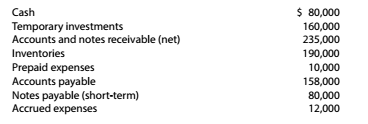Chapter 9, Problem 9.3.1P

Chapter
Section
Textbook Problem

Effect of transactions on current position analysis Data pertaining to the current position of Newlan Company are as follows:Instructions Compute (a) the working capital, (h) the current ratio, and (c) the quick ratio.

To determine

Concept Introduction:

Current Ratio:

Current Ratio is measure of the company's ability to pay off its current liabilities using its current assets. It is calculated by dividing the total current assets by total current liabilities. The formula of the current ratio is as follows:

Current Ratio=Current assetsCurrent liabilities

Acid test ratio:

Acid test ratio is also called Quick ratio. This ratio is calculated by dividing the quick assets (Cash, Cash equivalents, Short term investments and current receivables) by total current liabilities for the year. The formula for Acid test ratio is as follows:

Acid test ratio = (Cash + Cash equivalents + Short term investments + Accounts receivables) Current Liabilities

To Calculate:

The working capital, current ratio, and quick ratio.

Explanation

The working capital, current ratio, and quick ratio are calculated as follows:

 Current Assets: Cash $80,000 Temporary Investments$ 160,000 Accounts and Notes Receivables (net) $235,000 Total Quick Assets (A)$ 475,000 Add: Inventories $190,000 Add: Prepaid Expense$ 10,000 Total Current Assets (B) \$ 675,000

Still sussing out bartleby?

Check out a sample textbook solution.

See a sample solution

The Solution to Your Study Problems

Bartleby provides explanations to thousands of textbook problems written by our experts, many with advanced degrees!

Get Started